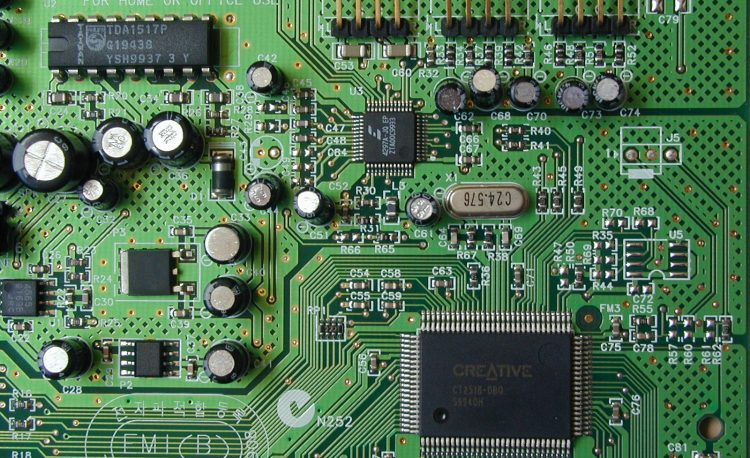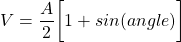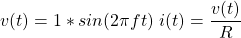STATUS: Coming# No Negatives in Physical Electronic Circuits & Physics Itself

(IN PROGRESS…)

I am an electrical engineer by trade. I have designed circuit boards all my life and never thought too much about the physics behind them. Now, using my Particle Model to interpret the physical reality of electronic components, I have come to realize that there are no negative numbers in physics.

## Light

Light is often described as an electromagnetic wave or a photon. The photon is a packet of energy with wave like properties. See Figure 1.

The waves, as seen in figure 1, show the electric and magnetic fields moving up then down or back and forth. And yet the equation for the waves use math from trigonometry and there is no up/down nor back/forth, just positives and negatives.

V = 5 * sin (angle) (1)

Sine values are positive in one half cycle and then negative in the next half cycle.

Using this math to solve a science problem seems to work. But there is nothing in Figure 1 that suggests a negative value. There is only up/down and back/forth. The amplitude of the UP half cycle has a value and the amplitude of the DOWN half cycle has a value. There is nothing negative about it.

## The Particle Model (TPM) for Light

The particle model for light is shown in figure 3.

TPM model for light is a stream of G1 particles moving at speed c. The number of particles can never be negative. The stream has many G1s at the peak and very few or no G1s in lowest part of the valley. The normal equation for the sine wave (figure 1) cannot be used. Equation 2 is a better equation.The values for V start at A/2, then the peak value A, back to A/2, and finally to 0. There are no negative values.

## Interference Patterns

Interference patterns occur when two light waves are partially out of phase. The standard explanation uses the normal values for the sine wave and the values cancel out. See figure 4.

In the constructive pattern 4a, the positive peaks add [C = A + B] and the negative peaks add [(-C) = (-A) + (-b)]. In the destructive pattern 4b, the negative peak cancels out the positive peak and the value is zero. So, the question is what happened to the light. Did they destroy themselves?

## Alternating Current

In figure 5, the red dots are electrons and the arrows indicate the direction.

The value of the current is calculated using equation 2 an 3.## The Math

In both the constructive and destructive cases, the positive value of the middle waveform adds to the positive value of the top waveform (A + B = C). In the same manner, the negative value of the middle waveform is added to the negative value of the top waveform (-A + -B = -C).

## Math Vs. Physics

Anyone who has been taught math, even in elementary school, knows that there are negative numbers shown on the number line. There is zero in the middle, positive numbers on the right and negative numbers on the left.

You can easily see how adding and subtracting a value to any number, positive or negative, will move you to a new number. But there are two interpretations of positive and negative. First, the value of the number can be positive or negative. Second, the minus and plus sign indicate the operation to be done, that is, add or subtract. It seems that it all works properly, that is, you get the right answer.

Not all operations make sense. There is a book by Peter Erickson titled “The Nature of Negative Numbers”, published in 2017 by Xulon Press Elite. Peter Erickson devises his own numbering system called the “Veritable Numbering System” that resolves problems. So, here are two of them.

Multiplying (+2) times (+2) is the same as adding 2 to the (+2) number, hence moving from (+2) to the (+4) number.  Adding positive numbers move the value to the right. Adding negative numbers moves the value to the left.

We are told that (-2) times (-2) is also (+4). Try moving from the (-2) number by moving to the right using a (-2) value and somehow get to (+4). Can’t do it!

## Square Root

We start with the process of squaring a number. (+2) squared is (+4). To square a number, take the number and multiply it by itself.

The square root is a process that tries to find the one number that, when multiplied by itself, gives the value (+4).

But the square root of (+4) has two answers: (+2) and (-2). Engineers and scientists have to know the specific situation and can select the most appropriate answer.

What about the square root of (-4)? What is the one number? If you use (+2) or (-2) as the number, you do not get (-4). The only answer is (-2) times (+2) which gives (-4). But (-2) and (+2) are not the same number and this violates the definition of what a square root is.

## Sine Wave and Equation:

Math has played a very strong roll in science. So much so, that Einstein suggested that physics would become a part of geometry. Using math as the main tool for science, results in trusting the math over logic, over common sense, and reason. Black holes were invented because the math of General Relativity predicts black holes. If you trust the math, then black holes become real to you. If you apply common sense to the prediction of black holes, then the singularity (infinite mass at the center of the black hole) is non-sense.

## Reducing Equations

Engineers and scientists love to reduce equations to simplify their use.

It’s time to take the position that the math must fit the physics.